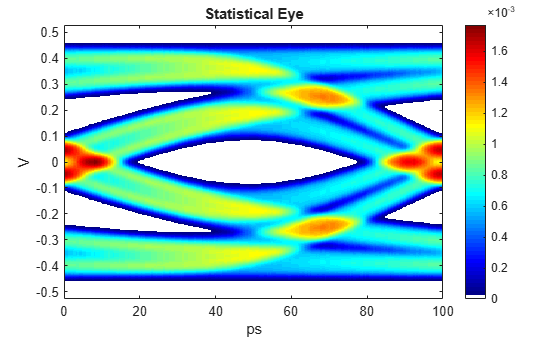# pulse2stateye

Statistical eye from pulse response

## Syntax

``[S,VH,TH] = pulse2stateye(P,N,M)``
``[___,S1] = pulse2stateye(P,N,M)``
``pulse2stateye(P,N,M)``

## Description

example

````[S,VH,TH] = pulse2stateye(P,N,M)` calculates and plots the statistical eye `S` from a pulse response `P` with `N` samples per symbol and `M` levels of modulation.The statistical eye is generated by progressively accumulating the histogram of each vertical slice `VH` by perturbing the ideal cursor voltage by the inter-symbol interference (ISI) voltages defined by the pulse response.The function uses Hula Hoop algorithm.```
````[___,S1] = pulse2stateye(P,N,M)` also returns the symbol transition histograms and crosstalk histogram `S1`.```
````pulse2stateye(P,N,M)` calculates and plots the statistical eye to the current figure.```

## Examples

collapse all

Load the pulse response column matrix from a file.

`load('PulseResponseReflective100ps.mat');`

Use the nonreturn to zero (NRZ) modulation scheme.

`modulation = 2;`

Calculate the statistical eye.

`[stateye,vh,th] = pulse2stateye(pulse,SamplesPerSymbol,modulation);`

Visualize the statistical eye using a color map.

```cmap = serdes.utilities.SignalIntegrityColorMap; figure, imagesc(th*SymbolTime*1e12,vh,stateye) colormap(cmap) colorbar axis('xy') xlabel('ps') ylabel('V') title('Statistical Eye')```## Input Arguments

collapse all

Input pulse response, specified as a column matrix. The first column contains the primary pulse response and the subsequent columns (if any) contain the crosstalk pulse responses.

Data Types: `double`

Number of samples per symbol, specified as a positive integer scalar.

Data Types: `double`

Number of modulation levels, specified as a positive integer scalar. `M` defines the modulation scheme used in the statistical eye calculation.

• If `M` = 2, the modulation scheme is nonreturn to zero (NRZ).

• If `M` = 4, the modulation scheme is four-level pulse amplitude modulation (PAM4).

Data Types: `double`

## Output Arguments

collapse all

Statistical eye of the pulse response `P`, returned as a matrix.

Data Types: `double`

Vertical voltage histogram bin centers, returned as a vector.

Data Types: `double`

Horizontal time histogram bin centers, returned as a vector.

Data Types: `double`

Symbol transition histograms and crosstalk histogram, returned as a 3-D matrix.`S1` is used to calculate the statistical eye `S`.

Data Types: `double`

## Version History

Introduced in R2020a